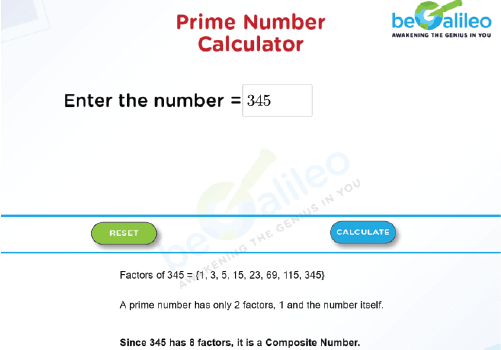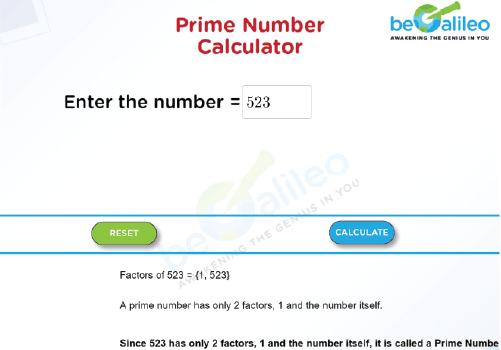>### Master Math with 1:1 Online Classes. Learn from Experts!# Prime Numbers Calculator

A Prime Number Calculator is an online tool for determining if a given number is a prime number or not. A number's factors can be found with this tool.

A Prime Number is any integer, or whole number, greater than 1 that is only divisible by 1 and itself. In other words, a prime number only has two factors, 1 and itself.

Prime Numbers are the building blocks of all positive integers, and any positive integer can be expressed as a product of primes in a unique way. Prime factorization is an important tool in number theory and cryptography.

We refer to a number as a composite number if it is not prime.

Composite numbers are whole numbers that have more than two factors.

## How to use the Prime Numbers Calculator?

Giving the input :
In the text box, enter the number you want to check to see if it is prime.

Calculation :
Click “CALCULATE”.
It will first display the factors and explain whether the given number is prime or composite.

Resetting the value :
Click “RESET”.

The previous calculations will be erased so that the new number can be entered.

## How to find whether a given number is prime.

Finding the factors :
Whenever a number is given, we have to find their factors.

Checking the number of factors :

• If the number of factors is 2, that is 1 and itself means, the given number is Prime.
• If the factors are greater than 2, then it is a Composite Number.

## Solved Examples

### Q1. Find whether the number 345 is prime or not.

Solution:
The factors of 345 are 1, 3, 5, 15, 23, 69, 115, and 345.
A prime number is a number that has only 2 factors, 1 and itself.
But 345 has 8 factors, which means it is a composite number.
Hence, 345 is a composite number.### Q2. Find whether the number 523 is prime or not.

Solution:
The factors of 523 are 1 and 523.
A prime number is a number that has only 2 factors, 1 and itself.
Hence, 523 is a prime number.